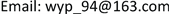1. 引言

2. 光学模型

I i λ = L i R i λ t + A i λ ( 1 − t i ) (1)

i 和 λ 分别表示像素的位置和RGB通道。 L i 表示入射光强度， R i λ 为场景反射率， A i λ 为局部大气光， t = e − β d i 为传输图， β 表示大气衰减系数， d i 表场景与相机之间的距离。式(1)中第一项和第二项分别称为直接投射光和环境光。分描述目标与反射光的衰减过程和成像路径上周围杂散光的累积过程。在夜间条件下，由于光照不均匀，为消除不均匀光照的影响。假设在大气中介质分布是均匀的，改写式(1)的到式(2)

I ^ i λ = R i λ t i + A ^ λ ( 1 − t i ) (2)

3. 去雾算法3.1. 算法框架

3.2. 输入图像生成3.2.1. 亮度图生成

L ( x ) = x + s i g m a _ s ⋅ g ( x ) − s i g m a _ h ⋅ g ( 1 − x ) g ( x ) = x c 1 exp ( − c 2 x ) (3)

3.2.2. 细节图生成

I i λ = J i j t + A i λ ( 1 − t i ) ，在一个局部块 Ω ，根据上述观察，引入局部均值 M ( x , y ) 根据大气光散射模型用 V i ( x , y ) 代替 A i ( 1 − t ( x , y ) ) ，然后改写退化模型。

M I ( x , y ) = M J ( x , y ) t ( x ) + V ( x , y ) (4)

V ( x , y ) = max ( min ( ρ B ( x , y ) , M I ( x , y ) ) , 0 ) (5)

M ¯ ( x , y ) = min ( x , y ) ∈ Ω ( K ( x i , y j ) ) (6)

S ( x i , y j ) = max ( I l ( x i , y j ) ) − min ( I l ( x i , y j ) ) (7)

t ( x , y ) = 1 − V ( x , y ) A Ω (8)

J ( x , y ) = I ( x , y ) − A Ω max ( t ( x , y ) , ε ) + A Ω (9)

3.3. 权重图

W L k = 1 / 3 [ ( R k − L k ) 2 + ( G k − L k ) 2 + ( B k − L k ) 2 ] (10)

W C k = exp ( − ( S k ( x ) − S max k ) 2 2 σ 2 ) (11)

V ( p ) = ∑ ∀ q ∈ I G ( p , q ) (12)

G ( p , q ) = | I p − I q | (13)

V ( p ) = | I p − I 1 | + | I p − I 2 | + ⋅ ⋅ ⋅ + | I p − I M | (14)

M为图像中像素点的个数，根据式(13)和式(14)，当像素点值相同时，它们的显著性值相同。以灰色图像为例，对于图像I中的强度 I p ，计算相对应的显著性值，式(15)。

V ( I p ) = ∑ j = 0 L − 1 N j G ( I p , j ) (15)

F ( x ) = ∑ W ¯ k ( x ) I k ( x ) (16)

4. 实验结果与分析

4.1. 本文算法结果

4.2. 主观评价分析

4.3. 客观评价分析

1) NESS梯度结构相似度。ChunLing Yang等  根据结构相似的思想结合人眼视觉系统的相关设计的。步骤如下：先为待评价图像制造参考图像。定义待评价图片I，参考图像使用 I r = L P F ( I ) ，即对待评价图像使用低通滤波得到参考图像。选择使用基于圆盘模型的均值滤波器和高斯模型的平滑滤波器都可以。为了更好的与成像系统匹配，建议使用 7 × 7 高斯平滑滤波。但是在实际处理中使用同等大小的均值滤波器并不会使评价效果下降很大。之后提取带评价图像I和参考图像 I r 的梯度信息。利用人眼对水平方向和垂直方向的边缘最为敏感的特性，使用Sobel算子分别提取两幅图片的梯度图像 G 和 G r 。最后将图像和 G r 划分成 8 × 8 的小块，快间步长为4，即相邻重复率50%。计算每块的，方差越大表面梯度

N R S S = 1 − 1 N ∑ i = 1 N S S I M ( x i , y i ) (17)

2) EAV点锐度。Ni. J等  提出一种基于边缘锐度算法来评价图像清晰度。通过统计图像某一方向的灰度变化来评价。公式入下：

D ( f ) = ∑ a b ( d f / d x ) 2 | f ( b ) − f ( a ) | (18)

E A V = D ( f ) = ∑ x , y ∑ i = 1 8 | d f / d x | M × N (19)

3) 基于自然场景统计的无参考图像质量评价算法(NIQE)。A. Mittal等  提出了基于自然场景统计的无参考图像质量评价算法，通过归一化的局部亮度系数提取多种可能反应图像质量的自然图像特征(文献  中详细介绍了所提取的特征)，并使用多元高斯模型(MVG)来拟合这些特征。文章中指出未退化图像的归一化亮度系数是符合高斯分布的，而退化图像(不管退化类型)不满足这种分布。所以，对于输入的评价图像，其MVG模型越接近自然图像的MVG模型，其图像的质量越高。自然图像的MVG模型是通过统计图像库中大量的自然为退化图像得到的。公式如下所示：

D ( v 1 , v 2 , ∑ , ∑ ) = ( v 1 − v 2 ) T ( ∑ 1 + ∑ 2 2 ) − 1 ( v 1 − v 2 ) (20)

Comparison with the NESS values of result
PeiZhangLiZhaoOur’s
Bridge0.70340.82340.81510.82550.8403
Street0.67470.73450.75870.80840.8799
Bus0.74480.76140.79530.88290.8593
Pavilion0.65320.78070.82010.89170.8709
Lane0.67120.70790.83820.86680.8812

Comparison with the EVA values of result
PeiZhangLiZhaoOur’s
Bridge72.9581.3482.2982.5584.03
Street68.4174.1579.3785.8489.45
Bus73.2575.8477.5382.2984.93
Pavilion67.3779.1381.3189.1787.09
Lane66.5974.2983.8280.1886.12

Comparison with the NIQE values of result
PeiZhangLiZhaoOur’s
Bridge4.78624.52344.21513.95253.9403
Street5.74755.13455.05874.80844.5799
Bus4.24913.76143.79533.18293.0532
Pavilion3.65323.08172.82012.89172.9709
Lane4.67124.27943.85823.46683.0812

5. 总结